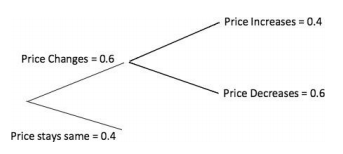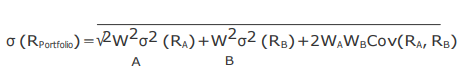# Quantitative Methods - Quantitative Methods Section 2

>>>>>>>>Quantitative Methods Section 2

• A

A continuous probability distribution.• B

A discrete probability distribution.• C

A normal distribution.• Option : B
• Explanation : When a dice is rolled, since there are a finite number of outcomes, it is an example of a discrete probability distribution. The continuous uniform distribution is defined over a range from a lower limit ‘a’ to an upper limit ‘b’. A normal distribution is symmetrical and bell-shaped.

• A

Cumulative distribution function.• B

Probability density function.• C

Probability function.• Option : A
• Explanation : The cumulative distribution function gives the probability that a random variable X is less than or equal to a particular value x, P (X < x). Probability function specifies probability that random variable takes on a specific value. Probability density function is used for continuous random variables.

 Net Profit (€) Probability Net profit of 0 0.25 Net profit of 2 or less 0.50 Net profit of 4 or less 0.75 Net profit of 6 or less 1.00

• A

1.00.• B

0.75.• C

0.50.• Option : C
• Explanation : P(X ≤ 6) = 1.0 and P(X ≤ 2) = 0.50. Therefore, P (2 ≤ X ≤ 6) = 1.0 – 0.50 = 0.50.

• A

0.24.• B

0.36.• C

0.40• Option : B
• Explanation : Consider the tree diagram below: The probability of a price decrease is equal to the probability of a price change times the probability of a decrease given a change = 0.6 * 0.6 = 0.36.The probability of a price decrease is equal to the probability of a price change times the probability of a decrease given a change = 0.6 * 0.6 = 0.36.

 Fund A Fund B Portfolio weights (%) 45 55 Expected returns (%) 23 13 Standard deviations (%) 14 6 Correlation between the returns ofFund X and Fund Y 0.7

The portfolio standard deviation of the returns is closest to:

• A

9.50%.• B

8.90%.• C

6.00%.• Option : B
• Explanation : The portfolio standard deviation of the returns is calculated through following formula:And covariance is calculated through following formula: Cov(RARB)=ρ (RARB) σ (RA)σ(RB)
First calculate the covariance, Cov= 0.7 ∗ .14 ∗ .06 = 0.00588, then enter values in the formula 1 for calculating portfolio standard deviation, you should get portfolio standard deviation = 8.90%.